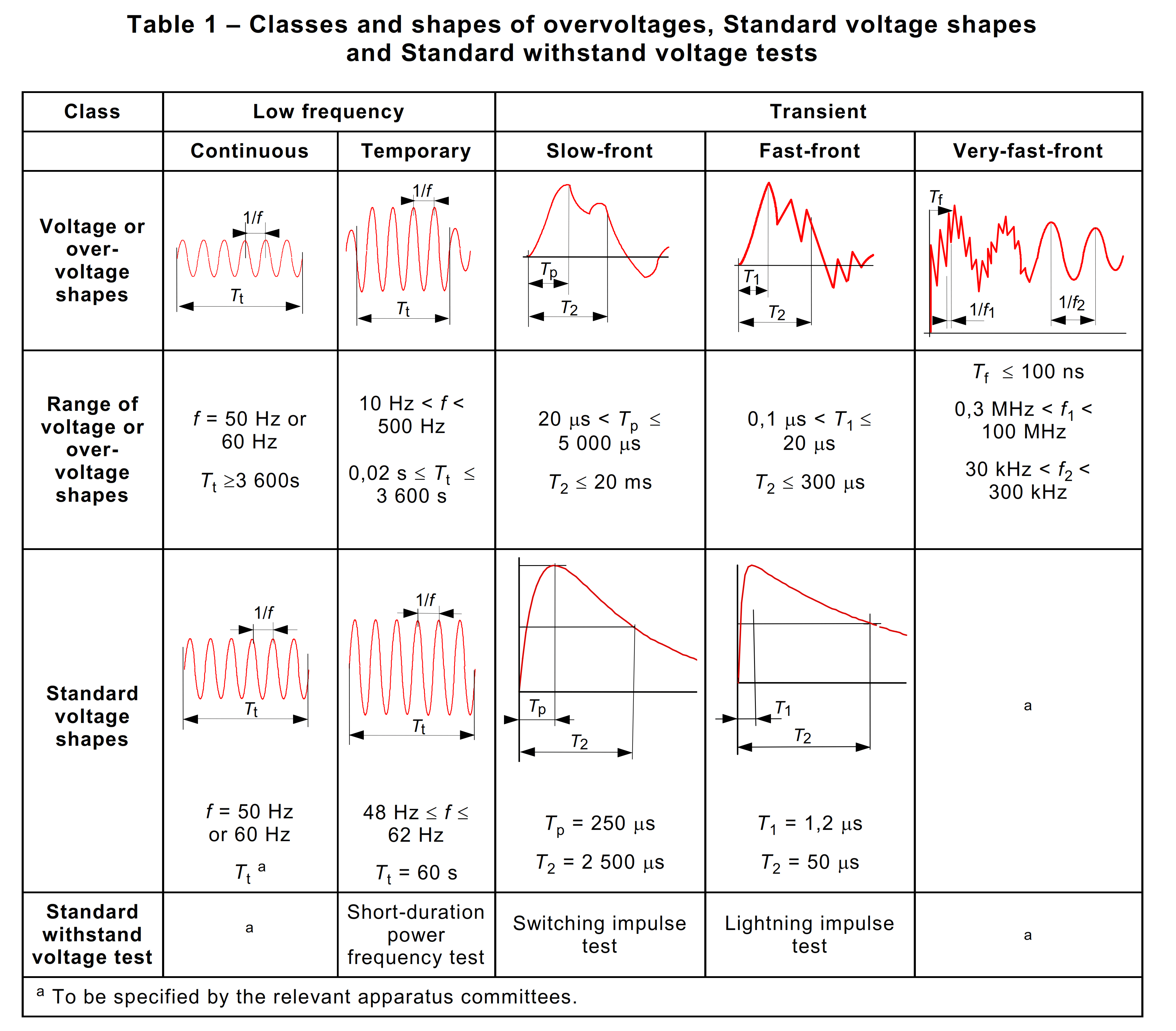Power frequency withstand is one of the criteria in the selection of electrical equipment rating. The selection of the appropriate power-frequency withstand level is related to the earth fault factor for the system. IEC 60071-1 [3.15] defines earth-fault factor k to be

... at a given location of a three-phase system and for a given system configuration, the ratio of the highest r.m.s phase-to-earth power-frequency voltage on a healthy phase during a fault to earth affecting one or more phases at any point on the system to the r.m.s value of phase-to-earth power-frequency voltage which would be obtained at the given location in the absence of any such fault ...

The earth-fault factor is used to determine the highest voltage electrical equipment can withstand during overvoltages such as lightning or switching surges.

IEC 60071-1 [3.17]classify voltages and overvoltages according to their shape and duration. Voltages and overvoltages are divided into the following classes:The earth fault factor can be calculated from the symmetrical component parameters of the system R0 , X0 , and X1 , where:
R0 is the zero phase sequence resistance
X0 is the zero phase sequence reactance
X1 is the positive phase sequence reactance

1. For a solidly earthed system for whichandwhere the earth fault factor will not exceed 1.4.
2. For a non-effectively earthed system for whichandwhere the earth fault factor will beor 1.7.
3. For a system earthed via an arc suppression coil the earth fault factor will be 1.9

The higher the earth fault factor, the higher should be the rated power-frequency one minute withstand voltage. Lightning impulse withstand voltage is tied to power-frequency withstand voltage so that once the latter has been determined the former is automatically fixed.

References:

1. IEC 60071-2011 Insulation co-ordination
2. The J & P Transformer Book, 12th Ed.

Categories:

Tags: